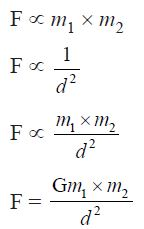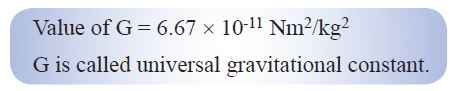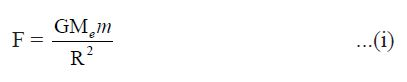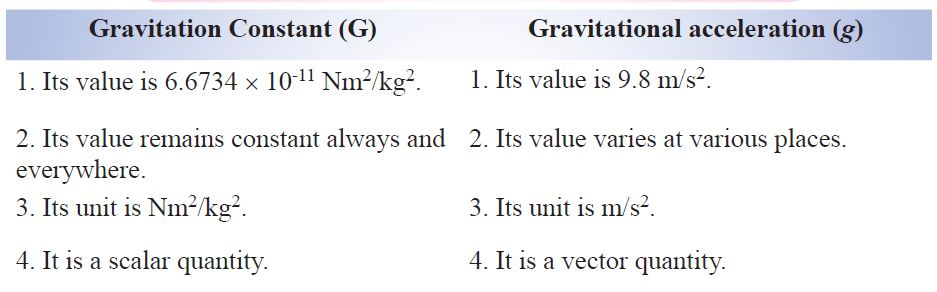# CBSE Class 9 Physics GRAVITATION## Gravitational Force of Earth

If we release a small stone without pushing it from a height, it accelerates towards earth. The stone is when accelerated towards earth, means some force is acting on it.

The force which pulls the objects towards the centre of the earth is known as gravitational force of the earth.

Here, stone also attracts earth. It means every object in universe attracts every other object.## Newton’s Universal Law of Gravitation

Sir Isaac Newton in 1687 proposed a law about the force of attraction between the two objects in the universe which is known as Newton’s law of gravitation.According to this law :

Every mass in this universe attracts every other mass with a force which is directly proportional to the product of two masses and inversely proportional to the square of the distance between them.

Let masses (m1) and (m2) of two objects are distance (d) apart, then force of attraction (F) between themwhere G is a constant and is known as Gravitational constant.If unit of F is in Newton, m is in kg, d is in metre, then unit of G can be calculated as :## Relation between Newton’s third law of motion and Newton’s law of gravitation

According to Newton’s third law of motion, “Every object exerts equal and opposite force on other object but in opposite direction.”

According to Newton’s law of gravitation, “Every mass in the universe attracts the every other mass.”

In case of freely falling stone and earth, stone is attracted towards earth means earth attracts the stone but according to Newton’s third law of motion, the stone should also attract the earth and really it is true that stone also attracts the earth with the same force F = m × a but due to very less mass of the stone, the acceleration (a) in its velocity is 9.8 m/s^2 and acceleration (a) of earth towards stone is 1.65 × 10^-24 m/s^2 which is negligible and we cannot feel it.

Importance of universal law of gravitation

(i) The force that binds us to the earth.
(ii) The motion of moon around the earth.
(iii) The motion of earth around the sun.
(iv) The tides due to moon and the sun.

Free fall of an object and acceleration during free fall

When an object is thrown upward, it reaches certain height, then it starts falling down towards earth. It is because the earth’s gravitational force exerts on it.

This fall under the influence of earth is called ‘free fall of an object’.

During this free fall direction do not change but velocity continuously changes which is called acceleration due to gravity.

It is denoted by ‘g’.

Its unit is same as acceleration m/s^2.

Gravitational Acceleration and its value at the surface of earth

The uniform acceleration produced in a freely falling object due to the gravitational force of earth, is called acceleration due to gravity. It is represented by ‘g’ and it always acts towards the centre of the earth.

Value of ‘g’ on the surface of earth

The force acting on an object ism = Mass of an object

and if acceleration due to gravity is ‘g’ due to force F then,

F = m × g ………………….. (ii)## Relationship and difference between ‘G’ and ‘g’

G = Gravitational constant
g = Acceleration due to gravity## Difference between G (Gravitational constant) and g (Acceleration due to gravity)Example. If two stones of 150 gm and 500 gm are dropped from a height, which stone will reach the surface of earth first and why ? Explain your answer.

Ans. It was Galileo, who first time demonstrated and depicted that the acceleration of an object falling freely towards earth does not depend on the mass of the object.

It can be verified by universal law of gravitation. Let an object of mass m, is allowed to fall from a distance of R, from the centre of the earth.So, acceleration in an object falling freely towards earth depends on the mass of earth and height of the object from the centre of the earth. So stones of mass 150 gm and 500 gm will reach the earth surface together.

Equation of motion when an object is falling freely towards earth or thrown vertically upwards :

Case 1. When an object is falling towards earth with initial velocity (u), then

Velocity (v) after t seconds, v = u + gt

Height covered in t seconds, h = ut + ½gt^2

Relation between v and u when t is not mentioned : v^2 = u^2 + 2gh

Mass
The mass of a body is the quantity of matter contained in it. Mass is a scalar quantity which has only magnitude but no direction.

SI unit of mass is kilogram which is written in short form as kg.
• Mass of a body is constant and does not change from place to place.
• Mass of a body is usually denoted by the small ‘m’.
• Mass of a body cannot be zero.

Weight
The force with which an object is attracted towards the centre of the earth, is called the weight of the object.

Force = m × a

In case of earth, a = g
So, F = m× g

But the force of attraction of earth on an object is called its weight (W). So, W = m × g

So, weight is the force and is SI unit is Newton (N). It depends on ‘g’ and is a vector quantity.

Relation between 1 kg wt and express it into Newton :

We know that W = m × g
If mass (m) = 1 kg, g = 9.8 m/s^2, then
W = 1 kg × 9.8 m/s^2
Or 1 kg wt = 9.8 N
So, the gravitational force of earth that acts on an object of mass 1 kg is called as 1 kg wt.

## Distinguish between Mass and WeightAnnouncements

Join our Online JEE Test Series for 499/- Only (Web + App) for 1 Year

Join our Online NEET Test Series for 499/- Only for 1 Year